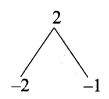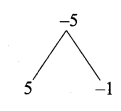# Chapter 1 Relations and Functions, Unit Exercise 1.

22 Nov 2020 7:45 pm

## Tamilnadu, Samacheer Kalvi, 10th, Maths, Solutions, Chapter 1, Relations and Functions, Unit, Exercise 1,

Question 1.

If the ordered pairs (x2 – 3x, y2 + 4y) and (-2, 5) are equal, then find x and y.
(x2 – 3x, y2 + 4y) = (-2, 5)
x2 – 3x = -2
x2 – 3x + 2 = 0
(x – 2) (x – 1) = 0
x – 2 = 0 or x – 1 = 0
x = 2 or 1y2 + 4y = 5
y2 + 4y – 5 = 0
(y + 5) (y – 1) = 0
y + 5 = 0 or y – 1 = 0
y = -5 or y = 1
The value of x = 2, 1
and 7 = -5, 1Question 2.
The Cartesian product A × A has 9 elements among which (-1, 0) and (0, 1) are found. Find the set A and the remaining elements of A × A.
Solution:
A = {-1, 0, 1}, B = {1, 0, -1}
A × B = {(-1, 1), (-1, 0), (-1, -1), (0, 1), (0, 0), (0, -1), (1, 1), (1, 0), (1, -1)}

Question 3.
Given that f(x) = {x−1−−−−−√4x≥1x<1.
Find
(i) f(0) (ii)f (3) (iii) f(a + 1) in terms of a.(Given that a > 0)
f(x) = x−1−−−−−√ ; f(x) = 4
(i) f(0) = 4
(ii) f(3) = 3−1−−−−√ = 2–√
(iii) f(a + 1) = a+1−1−−−−−−−−√ = a−−√

Question 4.
Let A = {9, 10, 11, 12, 13, 14, 15, 16, 17} and let f: A → N be defined by f(n) = the highest prime factor of n ∈ A. Write f as a set of ordered pairs and find the range of f.
Solution:
A = {9, 10, 11, 12, 13, 14, 15, 16, 17}
f: A → N
f(n) = the highest prime factor of n ∈ A
f = {(9, 3), (10, 5), (11, 11), (12, 3), (13, 13), (14, 7), (15, 5), (16, 2), (17, 17)}
Range = {3, 5, 11, 13, 7, 2, 17}
= {2, 3, 5, 7, 11, 13, 17}

Question 5.
Find the domain of the function

Domain of f(x) = {-1, 0, 1}

Question 6.
If f(x)= x2, g(x) = 3x and h(x) = x – 2 Prove that (fog)oh = fo(goh).
Solution:
f(x) = x2
g(x) = 3x
h(x) = x – 2
(fog)oh = x – 2
LHS = fo(goh)
fog = f(g(x)) = f(3x) = (3x)2 = 9x2
(fog)oh = (fog) h(x) = (fog) (x – 2)
= 9(x – 2)2 = 9(x2 – 4x + 4)
= 9x2 – 36x + 36 ……………. (1)
RHS = fo(goh)
(goh) = g(h(x)) = g(x – 2)
= 3(x – 2) = 3x – 6
fo(goh) = f(3x – 6) = (3x – 6)2
= 9x2 – 36x + 36 ………….. (2)
(1) = (2)
LHS = RHS
(fog)oh = fo(goh) is proved.

Question 7.
Let A= {1,2} and B = {1,2,3,4}, C = {5,6} and D = {5,6,7,8}. Verify whether A × C is a subset of B × D?
Given A = {1, 2}
B = {1, 2, 3, 4}
C = {5,6}
D = {5,6, 7,8}
A × C = {1,2} × {5,6}
= {(1,5) (1,6) (2, 5) (2, 6)}
B × D = {1,2, 3, 4} × {5, 6, 7, 8}
= {(1,5) (1,6) (1,7) (1,8)
(2, 5) (2, 6) (2,7) (2, 8)
(3, 5) (3, 6) (3, 7) (3, 8)
(4, 5) (4, 6) (4, 7) (4, 8)}
∴ A × C ⊂ B × D
Hence it is verified

Question 8.
If f(x) = x−1x+1,x≠1 Show that
f(f(x)) = – 1x, Provided x ≠ 0.

Question 9.
The functions f and g are defined by f{x) = 6x + 8; g(x) = x−23
(i) Calculate the value of gg $\frac { 1 }{ 2 }$
(a) Write an expression for gf (x) in its simplest form.
f(x) = 6x + 8 ; g(x) = x−23

Question 10.
Write the domain of the following real functions

(ii) if p(x) = =−54×2+1
p(x) is defined for all values of x. So domain is x ∈ R.

#### VISITORS COUNTUsers Today : 108Total Users : 120061Views Today : 543Total views : 468202